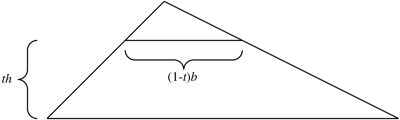# Barycenter of a triangle

created: 2022-08-06T19:50:01 (UTC -04:00)
tags: []
source: https://math.stackexchange.com/questions/1423664/barycenter-of-a-triangle

# linear algebra - Barycenter of a triangle

The barycenter, or centroid, of a triangle happens to be the mean of the three vertices, but the definition is the center of mass of the whole triangle.

To see why the barycenter is the mean of the vertices, consider a triangle with two vertices on the xx-axis:The mean of the yy-position would be

\frac{\int_0^1\overbrace{{\ \ \ \ }th{\ \ \ \ }}^y\,\overbrace{(1-t)b\,h\,\mathrm{d}t}^{\mathrm{d}A}}{\int_0^1\underbrace{(1-t)b\,h\,\mathrm{d}t}_{\mathrm{d}A}}
=\frac{bh^2\int_0^1t(1-t)\,\mathrm{d}t}{bh\int_0^1(1-t)\,\mathrm{d}t}
=\frac13h

That is, the distance from the side opposite each vertex to the barycenter is \frac13\frac13 the distance of the vertex from the side opposite.

Thus, the barycentric coordinates of the center of mass would be

M=\frac13A+\frac13B+\frac13C

Writing this as offsets from a point OO, we get

OM=\frac13OA+\frac13OB+\frac13OC